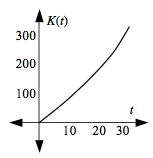### Home > APCALC > Chapter 6 > Lesson 6.4.3 > Problem6-153

6-153.

The function $k(t) = 8 - \cos(t) + 0.01t^2 + 0.01t$ helped Kira determine the rate at which electricity was being used for any day $t$ of the month. However, to manage total electrical production, Kira needs to determine a function that will accumulate the energy used over the course of the month. That is, she needs a function $K(t)$ that will determine the total energy used from the beginning of the month to time $t$.

1. If time $t = 0$, no electricity has been used. Write an equation for $K\left(t\right)$ that can be used to calculate the amount of electricity that has accumulated as a function of time. What is the relationship between the equations for $K(t)$ and $k(t)$?

Notice that lower-case, $k\left(t\right)$, is a rate function.

2. Use your equation for $K\left(t\right)$ to calculate the total amount of electricity used during this month.

Assume the month has $30$ days.

'Total electrical consumption' is another way to say 'accumulated power.'

3. Use your calculator to examine the graph of $y = K(t)$ for this $30$-day period. Then calculate the average amount of electricity used per day during this month. Does you answer match that in problem 6-144? How is this rate represented geometrically on the graph?

Make sure that this graph agrees with your function for $K\left(t\right)$.